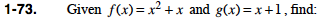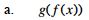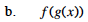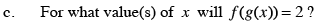### Home > PC > Chapter 1 > Lesson 1.2.1 > Problem1-73

1-73.
1. Given f(x) = x2 + x and g(x) = x + 1, find: Homework Help ✎

1. g(f(x))

2. f(g(x))

3. For what value(s) of x will f(g(x)) = 2?Substitute (x2 + x) for every x in the g function.Substitute (x + 1) for every x in the f function.x2 + 3x +2 = 2

Now set the equation equal to 0 and solve.